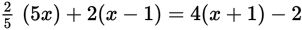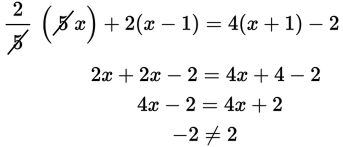# SAT Math Multiple Choice Question 490: Answer and Explanation

### Test Information

Question: 490

10. If, what is the value of x?

• A. x = -2
• B. x = 2
• C. There is no value of x for which the equation is true.
• D. There are infinitely many values of x for which the equation is true.

Explanation:

C

Difficulty: Medium

Category: Heart of Algebra / Linear Equations

Strategic Advice: Use the distributive property to simplify each of the terms that contains parentheses. Then use inverse operations to solve for x.